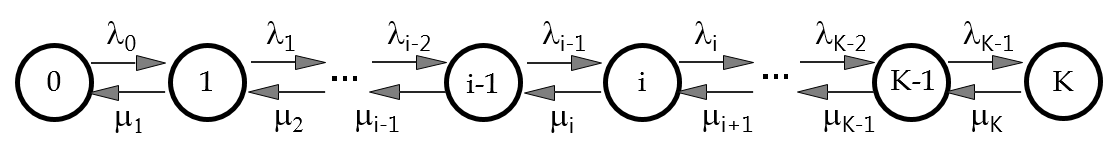# M(x)/M/K System with State-dependent Arrival Rates¶

(c) Tobias Hossfeld (Aug 2021)

This script and the figures are part of the following book. The book is to be cited whenever the script is used (copyright CC BY-SA 4.0):
Tran-Gia, P. & Hossfeld, T. (2021). Performance Modeling and Analysis of Communication Networks - A Lecture Note. Würzburg University Press. https://doi.org/10.25972/WUP-978-3-95826-153-2

We consider a loss system with $K$ servers. If all $K$ servers are occupied, incoming arrivals are rejected. The interarrival times $A_i$ are negative exponentially distributed with rate $\lambda_i$ when the system is in state $[X=i]$ for $i=0,\dots,K$. The service time of a job follows an exponential distribution with rate $\mu$.

The state-dependent arrival rates are denoted with M(x) - or sometimes M$_x$ - in Kendall's notation: M(x)/M/K-0

## Analysis of the System¶

The system is a Markovian system. To be more precise, we have a birth-and-death process, since transitions occur only between neighboring states. The state of the system is the number $X$ of jobs in the system.The transition rate $[X=i] \to [X=i+1]$ corresponds to the state-dependent arrival rate and we assume:

$\lambda_i = (i+1) \lambda$ for a given $\lambda$ and $i=0,\dots,K$.

Since there are $K$ servers, the service rate is

$\mu_i = i \mu$ für $i=1,\dots, K$.

### State Probabilities¶

The state probabilites are $P(X=i)=x(i)$.

The macro state equations are

$\lambda_{i-1} x(i-1) = \mu_{i} x(i)$ for $i=0,\dots K-1$.

We obtain the following state probabilities with the parameter $a = \lambda/\mu$:

$x(i) = \frac{\lambda_{i-1}}{\mu_i} x(i-1) = \frac{i \lambda}{i \mu} x(i-1) = a \cdot x(i-1) = a^i x(0)$

The state probability for the empty system $x(0)$ follows accordingly:

$1 = \sum_{i=0}^K x(i) = \sum_{i=0}^K a^i x(0) = x(0) \sum_{i=0}^K a^i = x(0) \frac{1-a^{K+1}}{1-a} \quad \Rightarrow \quad x(0) = \frac{1-a}{1-a^{K+1}}$

## Blocking Probability¶

The PASTA property must not be applied here, since the arrival process is not a Poisson process (although the interarrival times are exponentially distributed, but with state-dependent arrival rates).

As a consequence, we need to derive the state probability $x_A(i)$ that an arriving customer finds the system in state $[X_A=i]$. Then, the blocking probability is

$p_B = x_A(K)$.

To this end, we use the strong law of large numbers for Markov chains.

$x_A(i) = \frac{\lambda_i \cdot x(i)}{\sum_{i=0}^K \lambda_i \cdot x(i)}$

Note that the denominator is the mean arrival rate $\bar{\lambda}$ of the system:

$\bar{\lambda} = E[\lambda] = \sum_{i=0}^K \lambda_i x(i) = \sum_{i=0}^K (i+1)\lambda a^i \frac{1-a}{1-a^{K+1}} = \lambda \left( \frac{2-a}{1-a}+K - \frac{K+1}{1-a^{K+1}} \right)$

Thus:

$x_A(i) = \frac{\lambda_i}{\bar{\lambda}} \cdot x(i)$

Finally, we obtain:

$p_B = x_A(K) = \frac{(K+1)\lambda}{\bar{\lambda}} x(K) = \frac{(a-1)^2 (K+1) a^K}{((a-1) K+a-2) a^{K+1}+1}$

## Mean Number of Customers in the System¶

Due to Little's law: $E[X] = (1-p_B)\bar{\lambda} \cdot E[B] = (1-p_B)\bar{\lambda} \cdot \frac{1}{\mu}$.

Alternatively: $E[X]=\sum_{i=0}^K i \cdot x(i) = \sum_{i=0}^K i \cdot a^i \cdot x(0) = x(0) \frac{a(Ka^{K+1}-(K+1)a^K+1)}{(1-a)^2} = \frac{a(Ka^{K+1}-(K+1)a^K+1)}{(1-a)(1-a^{K+1})}$